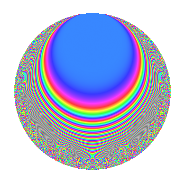Properties

 Label 19.4.eLevel 19 Weight 4 Character orbit e Rep. character $$\chi_{19}(4,\cdot)$$ Character field $$\Q(\zeta_{9})$$ Dimension 24 Newforms 1 Sturm bound 6 Trace bound 0

Related objects

Defining parameters

 Level: $$N$$ = $$19$$ Weight: $$k$$ = $$4$$ Character orbit: $$[\chi]$$ = 19.e (of order $$9$$ and degree $$6$$) Character conductor: $$\operatorname{cond}(\chi)$$ = $$19$$ Character field: $$\Q(\zeta_{9})$$ Newforms: $$1$$ Sturm bound: $$6$$ Trace bound: $$0$$

Dimensions

The following table gives the dimensions of various subspaces of $$M_{4}(19, [\chi])$$.

Total New Old
Modular forms 36 36 0
Cusp forms 24 24 0
Eisenstein series 12 12 0

Trace form

 $$24q$$ $$\mathstrut -\mathstrut 6q^{2}$$ $$\mathstrut -\mathstrut 3q^{3}$$ $$\mathstrut -\mathstrut 24q^{4}$$ $$\mathstrut -\mathstrut 6q^{5}$$ $$\mathstrut +\mathstrut 42q^{6}$$ $$\mathstrut +\mathstrut 3q^{7}$$ $$\mathstrut -\mathstrut 75q^{8}$$ $$\mathstrut -\mathstrut 51q^{9}$$ $$\mathstrut +\mathstrut O(q^{10})$$ $$24q$$ $$\mathstrut -\mathstrut 6q^{2}$$ $$\mathstrut -\mathstrut 3q^{3}$$ $$\mathstrut -\mathstrut 24q^{4}$$ $$\mathstrut -\mathstrut 6q^{5}$$ $$\mathstrut +\mathstrut 42q^{6}$$ $$\mathstrut +\mathstrut 3q^{7}$$ $$\mathstrut -\mathstrut 75q^{8}$$ $$\mathstrut -\mathstrut 51q^{9}$$ $$\mathstrut +\mathstrut 75q^{10}$$ $$\mathstrut +\mathstrut 39q^{11}$$ $$\mathstrut -\mathstrut 219q^{12}$$ $$\mathstrut -\mathstrut 156q^{13}$$ $$\mathstrut +\mathstrut 93q^{14}$$ $$\mathstrut -\mathstrut 192q^{15}$$ $$\mathstrut +\mathstrut 504q^{16}$$ $$\mathstrut +\mathstrut 12q^{17}$$ $$\mathstrut +\mathstrut 264q^{18}$$ $$\mathstrut +\mathstrut 546q^{19}$$ $$\mathstrut -\mathstrut 198q^{20}$$ $$\mathstrut +\mathstrut 453q^{21}$$ $$\mathstrut -\mathstrut 6q^{22}$$ $$\mathstrut +\mathstrut 6q^{23}$$ $$\mathstrut +\mathstrut 192q^{24}$$ $$\mathstrut -\mathstrut 498q^{25}$$ $$\mathstrut -\mathstrut 639q^{26}$$ $$\mathstrut -\mathstrut 870q^{27}$$ $$\mathstrut -\mathstrut 1368q^{28}$$ $$\mathstrut -\mathstrut 630q^{29}$$ $$\mathstrut -\mathstrut 522q^{30}$$ $$\mathstrut -\mathstrut 591q^{31}$$ $$\mathstrut +\mathstrut 147q^{32}$$ $$\mathstrut +\mathstrut 1506q^{33}$$ $$\mathstrut -\mathstrut 408q^{34}$$ $$\mathstrut +\mathstrut 2001q^{35}$$ $$\mathstrut +\mathstrut 1059q^{36}$$ $$\mathstrut -\mathstrut 72q^{37}$$ $$\mathstrut +\mathstrut 2934q^{38}$$ $$\mathstrut +\mathstrut 336q^{39}$$ $$\mathstrut +\mathstrut 2886q^{40}$$ $$\mathstrut -\mathstrut 477q^{41}$$ $$\mathstrut +\mathstrut 237q^{42}$$ $$\mathstrut +\mathstrut 588q^{43}$$ $$\mathstrut -\mathstrut 3423q^{44}$$ $$\mathstrut -\mathstrut 1569q^{45}$$ $$\mathstrut -\mathstrut 1728q^{46}$$ $$\mathstrut -\mathstrut 1242q^{47}$$ $$\mathstrut -\mathstrut 4599q^{48}$$ $$\mathstrut -\mathstrut 639q^{49}$$ $$\mathstrut -\mathstrut 1788q^{50}$$ $$\mathstrut +\mathstrut 9q^{51}$$ $$\mathstrut +\mathstrut 2733q^{52}$$ $$\mathstrut -\mathstrut 300q^{53}$$ $$\mathstrut +\mathstrut 3777q^{54}$$ $$\mathstrut +\mathstrut 315q^{55}$$ $$\mathstrut +\mathstrut 4638q^{56}$$ $$\mathstrut +\mathstrut 3342q^{57}$$ $$\mathstrut -\mathstrut 2820q^{58}$$ $$\mathstrut +\mathstrut 2097q^{59}$$ $$\mathstrut +\mathstrut 1116q^{60}$$ $$\mathstrut -\mathstrut 2316q^{61}$$ $$\mathstrut -\mathstrut 1320q^{62}$$ $$\mathstrut -\mathstrut 2979q^{63}$$ $$\mathstrut -\mathstrut 1785q^{64}$$ $$\mathstrut -\mathstrut 2433q^{65}$$ $$\mathstrut -\mathstrut 1590q^{66}$$ $$\mathstrut +\mathstrut 57q^{67}$$ $$\mathstrut -\mathstrut 438q^{68}$$ $$\mathstrut -\mathstrut 1767q^{69}$$ $$\mathstrut -\mathstrut 213q^{70}$$ $$\mathstrut -\mathstrut 792q^{71}$$ $$\mathstrut -\mathstrut 1686q^{72}$$ $$\mathstrut +\mathstrut 4068q^{73}$$ $$\mathstrut +\mathstrut 4287q^{74}$$ $$\mathstrut +\mathstrut 1332q^{75}$$ $$\mathstrut +\mathstrut 5538q^{76}$$ $$\mathstrut +\mathstrut 3786q^{77}$$ $$\mathstrut +\mathstrut 2121q^{78}$$ $$\mathstrut +\mathstrut 1824q^{79}$$ $$\mathstrut -\mathstrut 2739q^{80}$$ $$\mathstrut +\mathstrut 1536q^{81}$$ $$\mathstrut +\mathstrut 2205q^{82}$$ $$\mathstrut +\mathstrut 1071q^{83}$$ $$\mathstrut -\mathstrut 1437q^{84}$$ $$\mathstrut -\mathstrut 2394q^{85}$$ $$\mathstrut -\mathstrut 5256q^{86}$$ $$\mathstrut +\mathstrut 759q^{87}$$ $$\mathstrut +\mathstrut 1101q^{88}$$ $$\mathstrut -\mathstrut 3006q^{89}$$ $$\mathstrut -\mathstrut 3822q^{90}$$ $$\mathstrut -\mathstrut 3285q^{91}$$ $$\mathstrut -\mathstrut 1452q^{92}$$ $$\mathstrut -\mathstrut 135q^{93}$$ $$\mathstrut -\mathstrut 1086q^{94}$$ $$\mathstrut -\mathstrut 3078q^{95}$$ $$\mathstrut -\mathstrut 1590q^{96}$$ $$\mathstrut -\mathstrut 2535q^{97}$$ $$\mathstrut -\mathstrut 2403q^{98}$$ $$\mathstrut +\mathstrut 492q^{99}$$ $$\mathstrut +\mathstrut O(q^{100})$$

Decomposition of $$S_{4}^{\mathrm{new}}(19, [\chi])$$ into irreducible Hecke orbits

Label Dim. $$A$$ Field CM Traces $q$-expansion
$$a_2$$ $$a_3$$ $$a_5$$ $$a_7$$
19.4.e.a $$24$$ $$1.121$$ None $$-6$$ $$-3$$ $$-6$$ $$3$$